## If the heater can heat 200 mL of water from 22 ∘C to 91 ∘C in 13.9 min , approximately how much current does it draw from the car’s 12-V bat

Question

If the heater can heat 200 mL of water from 22 ∘C to 91 ∘C in 13.9 min , approximately how much current does it draw from the car’s 12-V battery? Assume the manufacturer’s claim of 75% efficiency. Express your answer using two significant figures.

in progress 0
2 weeks 2021-08-29T00:04:13+00:00 1 Answers 0 views 0

1. Explanation:

First, we will convert 200 ml into kg as follows.

200 ml = 0.2 kg

Now, we will take the density of water as 1 g/ml.

And,   dT =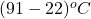=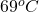Also, we know that specific heat of water is 4.186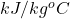. Therefore, we will calculate the heat energy as follows.

q =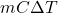=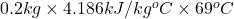= 5.77 kJ

Now, we will calculate the power delivered as follows.

P =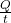=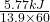= 69.1 W

It is given that efficiency is 75%. Therefore, input power will be calculated as follows.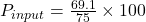= 92.13 W

Now, we will calculate the current as follows.

P =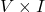I =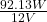= 7.67 A

Therefore, we can conclude that current drawn from the car’s 12-V battery is 7.67 A.